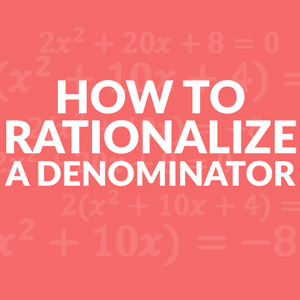##How to Rationalize the Denominator

Rationalizing the denominator is the process of moving any root or irrational number (cube roots or square roots) out of the bottom of the fraction (denominator) and to top of the fraction (numerator).

The denominator is the bottom part of a fraction. This part of the fraction can not have any irrational numbers. The most common used irrational numbers that are used are radical numbers, for example √3. Keep in mind that some radicals are actually rational numbers and can be simplified into a whole number. √9 = 3 is one example. So when rationalizing the denominator is used, it is turning irrational numbers into rational numbers.

The best way to do this is to multiply the radical by itself. This then turns it into a perfect square and can then be simplified. For example √3 x √3 = √3 x 3 = √9 = 3. When applying this to fractions you need to remember to make sure what you do to the denominator you have to do to the numerator in order to not change the value of the fraction. Remember, when you multiply by 1, you are not changing the value.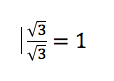This becomes more complicated when you have an expression as the denominator. You then need to multiply by the conjugate. The conjugate of 2 – √3 would be 2 + √3. You use the inverse sign in order to make sure there is no b term when you multiply the expressions.

## Steps to Rationalize the Denominator and Simplify

1. Multiply both the numerator and denominator by the radical that is in the denominator or by the conjugate.
2. Simplify

## Rationalizing the Denominator Examples

When there is only a radical in the denominator.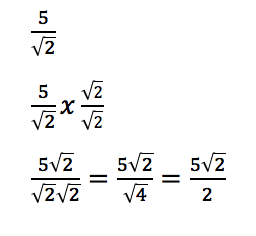Multiply the numerator and denominator by the radical that is in the denominator.

Simplify

When there is more than just a radical in the denominator.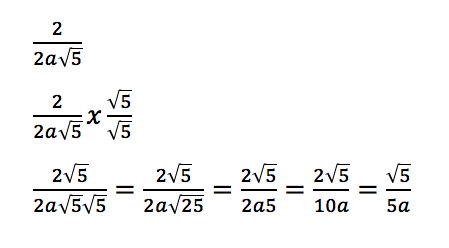Multiply the numerator and denominator by the radical that is in the denominator.

Simplify

When there are two terms in the denominator.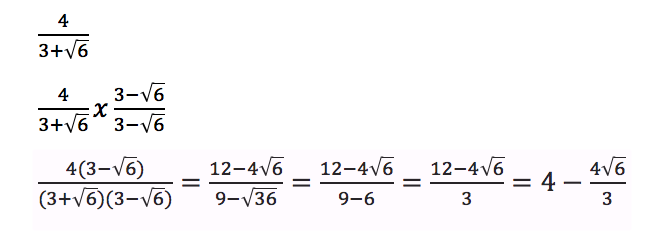Start by finding the conjugate. The conjugate of is .

Multiply the numerator and denominator by the conjugate.

Simplify

## Why is Rationalizing the Denominator Important in Math?

Often times radicals are seen with geometric concepts involving circles like finding the radius of a circle given the area, Pythagorean’s theorem, and distances formula.

In the modern world, calculators and other programs can do division of radicals easily, however, before calculators it would have been easier to divide a number by 3 and not .